2019-11-01 20:34:22 weixin_44225182 阅读数 741

## 题目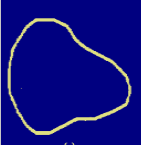## 概念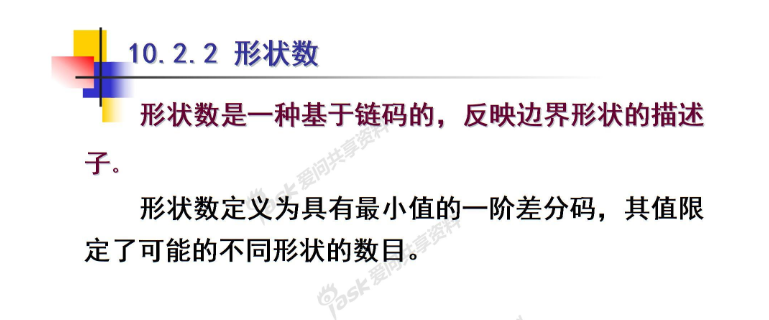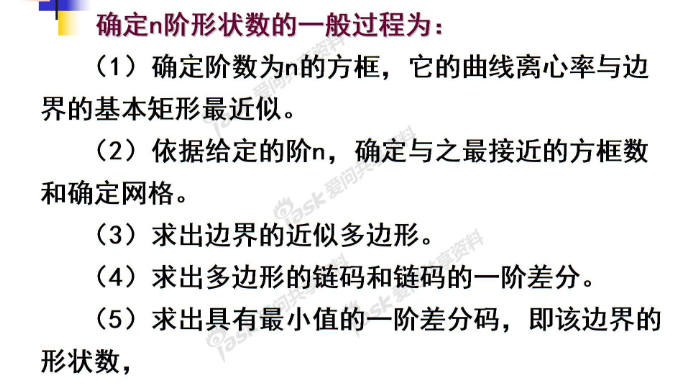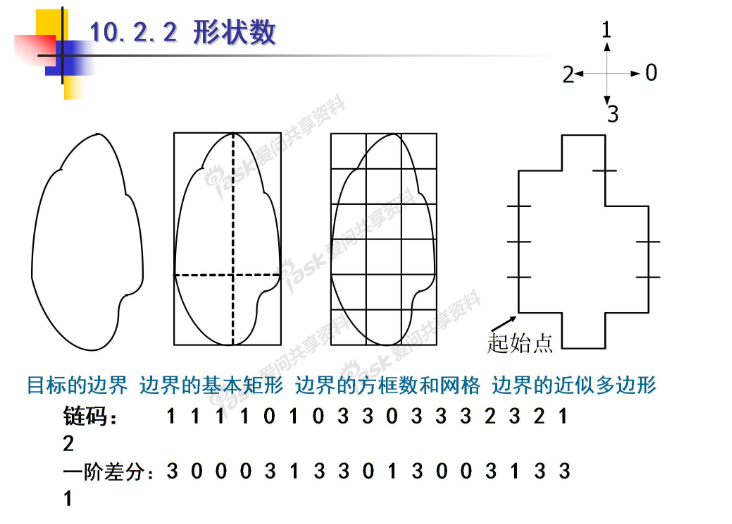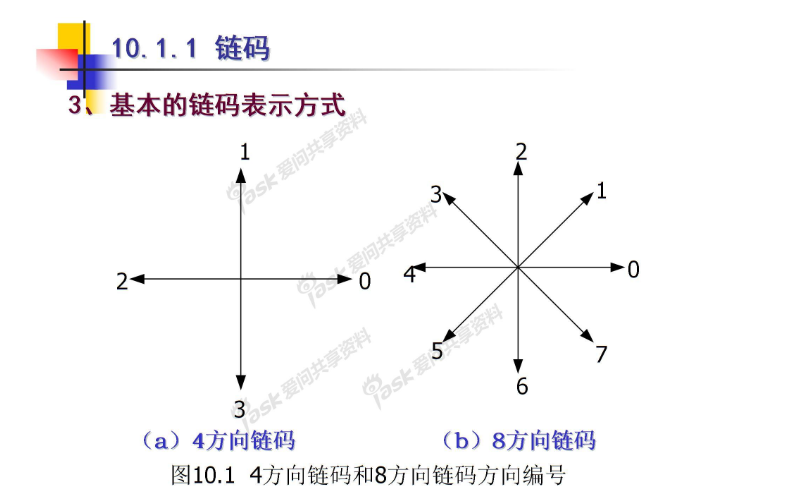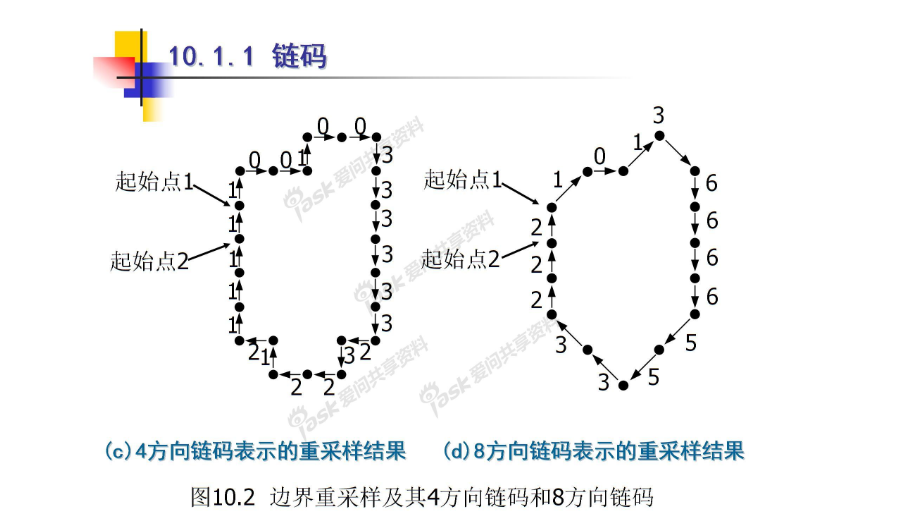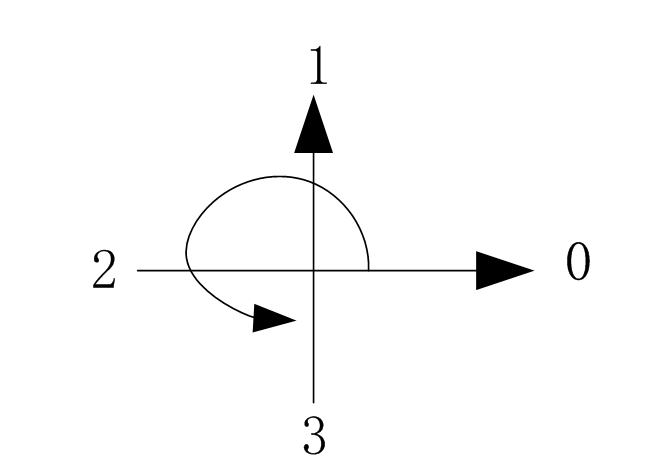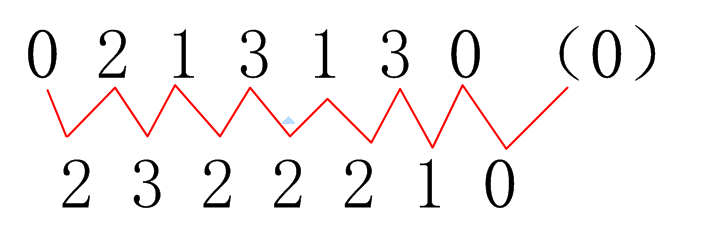C++算法（以4方向链码为例）

• 计算第一个数与第二个数差值
• 比较二者大小
• 若第一个数大，则一阶差分为 差值相反数
• 若第二个数大，则一阶差分为 4-差值

## 整理计算链码算法

1. 对图像进行预处理 ，比如去除噪声、边缘化等 依照不同图片采取不同措施
2. 进行重取样（重取样原理在下面代码中）
3. 随便找一个起始点，记录起始位置
4. 利用循环 or 递归 求链码（类似C++中的走迷宫）

## 实验代码

``````
% write by 海轰
%该程序针对特定图片其作用
%为测试程序

%过滤边缘白点 这里仅针对这幅图像
t(:,1)=0;
t(140:145,:)=0;

imshow(t),title('原图');

[m,n]=size(t);

%行扫描 找出图像白色区域最左边的端点
for i=1:m
x=0;y=141;
tem=t(i,:);
h=sum(tem);
if h~=0
for j=1:n
if tem(j)==1
x=j;
break
end
end

%行扫描 反转 寻找白点区域最右边的端点
tem=flip(tem);
for j=1:n
if tem(j)==1
y=n-j+1;
break;
end
end

%对区域进去填充 便于找到边界
for j=x+1:y-1
t(i,j)=1;
end
end
end

figure,imshow(t),title('填充区域后');
t=bwperim(t,8);
figure,imshow(t),title('找到边界');

a=t;
rt=zeros(145,141);

%对图像进行重取样
%假设原图像为100*100 一点为（62，73）
%重取样模板为100*100 但是间隔为10 （分成10*10）
%先对（62,73）/10=(6.2,7.3)
%再取整 （6,7）
%再还原 10*（6,7）=（60,70）
%这样重取样后得到（60,70）
for i=1:m
for j=1:n
if t(i,j)==1
if round(j/15)==0
rt(15*round(i/15),15*(round(j/15)+1))=1;
else
rt(15*round(i/15),15*round(j/15))=1;
end
end
end
end
figure,imshow(rt),title('重取样后');

stack=[0 0];%保存起点
code=[];%保存链码
points=zeros(25,2);%保存端点
k=0;
t=rt;

%随便寻找起点
for i=1:m
for j=1:n
if t(i,j)==1
stack=[i j];
break;
end
end
end

s1=stack(1);
s2=stack(2)+1;

%while循环求链码
while (s1~=stack(1)||s2~=stack(2))&&k<500
k=k+1;

if k==1
s2=s2-1;
end

if s2+15<=141
if t(s1,s2+15)==1
code(k)=0;
points(k,1)=s1;
points(k,2)=s2;
t(s1,s2)=0;
s1=s1;s2=s2+15;
continue;
end
end

if s1-15>0&&s2+15<=141
if t(s1-15,s2+15)==1
code(k)=1;
points(k,1)=s1;
points(k,2)=s2;
t(s1,s2)=0;
s1=s1-15;
s2=s2+15;
continue;
end
end

if s1-15>0
if t(s1-15,s2)==1
code(k)=2;
points(k,1)=s1;
points(k,2)=s2;
t(s1,s2)=0;
s1=s1-15;s2=s2;
continue;
end
end

if s1-15>0&&s2-15>0
if t(s1-15,s2-15)==1
code(k)=3;
points(k,1)=s1;
points(k,2)=s2;
t(s1,s2)=0;
s1=s1-15;s2=s2-15;
continue;
end
end

if s2-15>0
if t(s1,s2-15)==1
code(k)=4;
points(k,1)=s1;
points(k,2)=s2;
t(s1,s2)=0;
s1=s1;s2=s2-15;
continue;
end
end

if s2-15>0
if t(s1+15,s2-15)==1
code(k)=5;
points(k,1)=s1;
points(k,2)=s2;
t(s1,s2)=0;
s1=s1+15;
s2=s2-15;
continue;
end
end

if t(s1+15,s2)==1
code(k)=6;
points(k,1)=s1;
points(k,2)=s2;
t(s1,s2)=0;
s1=s1+15;s2=s2;
continue;
end

if s2+15<=141
if t(s1+15,s2+15)==1
code(k)=7;
points(k,1)=s1;
points(k,2)=s2;
t(s1,s2)=0;
s1=s1+15;
s2=s2+15;
continue;
end
end
end

xt=rt-t;
figure,imshow(xt),title('相似多边形'),hold on;
for i=1:25
if i==25
plot([points(25,2);points(1,2)],[points(25,1);points(1,1)]);
else
plot(points(i:i+1,2),points(i:i+1,1));
end
end
``````

## 结果图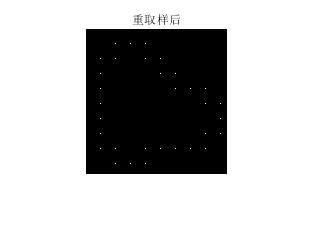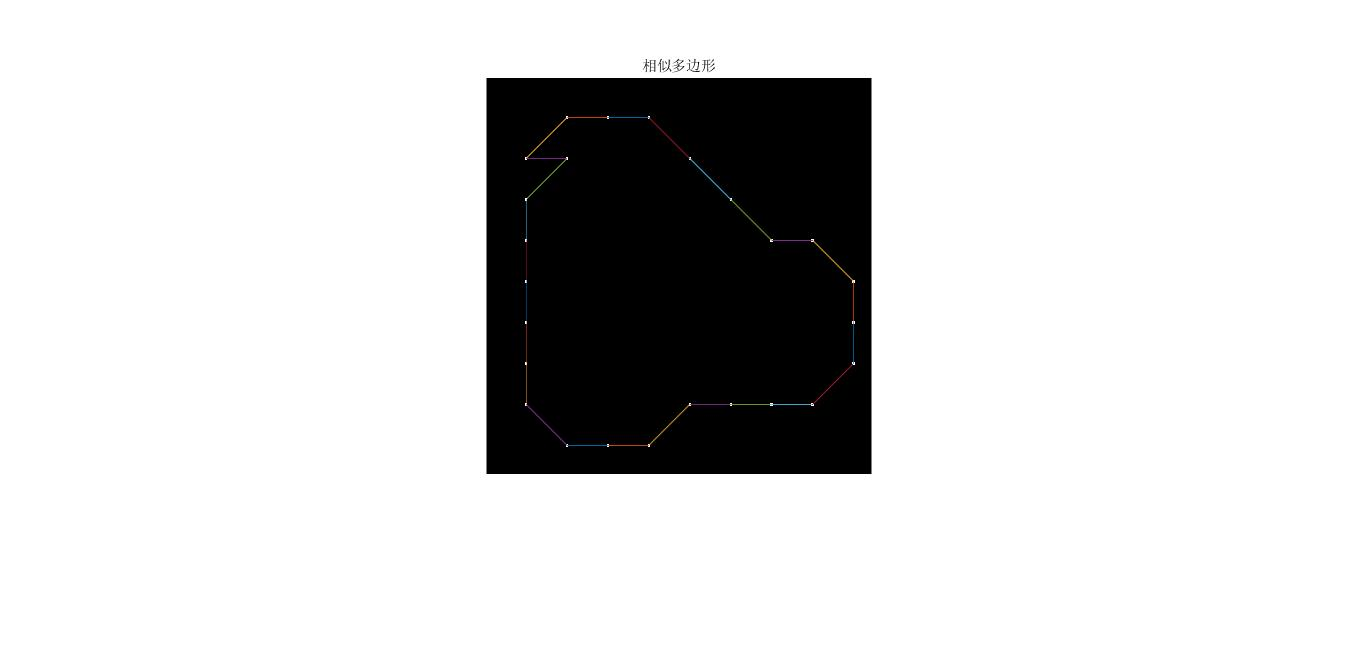## 更多

2017-12-31 11:59:48 coming_is_winter 阅读数 2369

链码（又称为freeman码）是用曲线起始点的坐标和边界点方向代码来描述曲线或边界的方法，常被用来在图像处理、计算机图形学、模式识别等领域中表示曲线和区域边界。它是一种边界的编码表示法，用边界方向作为编码依据，为简化边界的描述，一般描述的是边界点集。它将线状地物或区域边界，由起点和一系列在基本方向上的单位矢量，给出每个后续点相对其前继点的方向编码表示。如上图所示，在寻找链码时，制定一个起始点（黑点），沿着逆时针方向，寻找一圈直到闭合，链码为：300301121232，下一步的工作是将其归一化，这里归一化的具体指链码寻找具有旋转不变性和起始点选择随意性，，简单点的例子是001,010,100是起始点选择不同的一个链码的三种不同表现形式，这里统一将其归一化为代表的最小整数：001（四进制）。推广到上面的链码，归一化为：003011212323，集体左移一位。。下一步求导数：前一位链码与后一位的差分（这里指逆时针转动90°的倍数（四链接））。2012-02-11 15:58:54 renshengrumenglibing 阅读数 6627

```/************************************************************************/
/* 查找物体的边界，输出已排序的边界序列 适应于单一区域        */
/************************************************************************/
//若能够输出边界点的序列则是比较有用的
#include<cv.h>
#include <highgui.h>
#include <iostream>
#include <stack>
using namespace std;

int main(){
IplImage * image,*image2;
cvNamedWindow("image",1);
cvShowImage("image",image);

image2 = cvCreateImage(cvSize(image->width, image->height),image->depth,1);
cvZero(image2);//image2 赋值为0
//寻找区域的左上角点
CvPoint startPoint = cvPoint(0,0);
bool bFindStartpoint = false;
int i ,j;
unsigned char * ptr,*dst;
stack<int> board;//奇数位存储x坐标，偶数位存储y坐标

//当前扫描点
CvPoint currentPoint = cvPoint(0,0);
//邻域的8个点的方向
int directions = {{0,1},{1,1},{1,0},{1,-1},{0,-1},{-1,-1},{-1,0},{-1,1}};
int beginDirection = 0;
bool bFindBoardpoint = false;//寻找到邻域的边界点的判定
for (i = 0 ; i< image->height && bFindStartpoint == false; i++)
{
for (j = 0 ; j< image->width && bFindStartpoint == false; j++)
{
ptr = (unsigned char *)(image->imageData + i*image->widthStep + j);
if (*ptr == 255)
{
startPoint.x = j;
startPoint.y = i;
bFindStartpoint = true;
//cout<<"x:  " << j <<"y :  " <<i <<endl;
}
}
}

//进行边界跟踪 每次搜索8个方向的点 找到了即停止
currentPoint = startPoint;
bFindStartpoint = false;
beginDirection = 0;
board.push(startPoint.x);
board.push(startPoint.y);
while (!bFindStartpoint)
{
bFindBoardpoint = false;
//在8个方向寻找符合条件的边界点
while (!bFindBoardpoint)
{
//进行出界判定  不对啊 这张图不可能出界啊
ptr = (unsigned char *)(image->imageData + (currentPoint.y + directions[beginDirection])* image->widthStep + currentPoint.x + directions[beginDirection]);
if (*ptr == 255)
{
bFindBoardpoint = true;
currentPoint.x +=  directions[beginDirection];
currentPoint.y  += directions[beginDirection];
/************************************************************************/
/*  此处添加序列存储的代码                    */
/************************************************************************/
//一、将边界存储到图片中
dst  = (unsigned char *)image2->imageData + currentPoint.y * image2->widthStep + currentPoint.x;
*dst = 255;

//二、将边界点的序列存储到一个堆栈中
board.push(currentPoint.x);
board.push(currentPoint.y);

if (currentPoint.x == startPoint.x  && currentPoint.y == startPoint.y )
{
bFindStartpoint = true;
}
//改变下次首先开始扫描的方向
beginDirection -= 2;
if (beginDirection < 0)
{
beginDirection += 8;
}

}
else
{
beginDirection ++;
beginDirection = beginDirection%8;
}
}
//cout<<"currentPoint    "<<currentPoint.x <<"     "<< currentPoint.y<<endl;
}
cvNamedWindow("image2",1);
cvShowImage("image2",image2);

//显示堆栈中的数据 顺时针存储,逆时针显示
//注意：显示时候堆栈中已经没有数据了
/*	int x,y;
while(!board.empty())
{
y = board.top();
board.pop();
x = board.top();
board.pop();
cout<<"x   "<<x<<"    y    "<<y<<endl;
}
*/
cvWaitKey(0);
return 0;
}``````/************************************************************************/
/* 轮廓跟踪算法获取物体的轮廓序列 生成边界链码   */
/************************************************************************/
#include<cv.h>
#include <highgui.h>
#include <iostream>
#include <stack>
using namespace std;

int main(){
IplImage * image,*image2,*image3;
cvNamedWindow("image",1);
cvShowImage("image",image);

image2 = cvCreateImage(cvSize(image->width, image->height),image->depth,1);
image3 = cvCreateImage(cvSize(image->width, image->height),image->depth,1);
cvZero(image2);//image2 赋值为0
cvZero(image3);
//寻找区域的左上角点
CvPoint startPoint = cvPoint(0,0);
bool bFindStartpoint = false;
int i ,j;
unsigned char * ptr,*dst;
stack<int> board;//奇数位存储x坐标，偶数位存储y坐标

//当前扫描点
CvPoint currentPoint = cvPoint(0,0);
//邻域的8个点的方向
int directions = {{0,1},{1,1},{1,0},{1,-1},{0,-1},{-1,-1},{-1,0},{-1,1}};
int beginDirection = 0;
bool bFindBoardpoint = false;//寻找到邻域的边界点的判定
for (i = 0 ; i< image->height && bFindStartpoint == false; i++)
{
for (j = 0 ; j< image->width && bFindStartpoint == false; j++)
{
ptr = (unsigned char *)(image->imageData + i*image->widthStep + j);
if (*ptr == 255)
{
startPoint.x = j;
startPoint.y = i;
bFindStartpoint = true;
//cout<<"x:  " << j <<"y :  " <<i <<endl;
}
}
}

//进行边界跟踪 每次搜索8个方向的点 找到了即停止
currentPoint = startPoint;
bFindStartpoint = false;
beginDirection = 0;
board.push(startPoint.x);
board.push(startPoint.y);
while (!bFindStartpoint)
{
bFindBoardpoint = false;
//在8个方向寻找符合条件的边界点
while (!bFindBoardpoint)
{
//进行出界判定  不对啊 这张图不可能出界啊
ptr = (unsigned char *)(image->imageData + (currentPoint.y + directions[beginDirection])* image->widthStep + currentPoint.x + directions[beginDirection]);
if (*ptr == 255)
{
bFindBoardpoint = true;
currentPoint.x +=  directions[beginDirection];
currentPoint.y  += directions[beginDirection];
/************************************************************************/
/*  此处添加序列存储的代码                    */
/************************************************************************/
//一、将边界存储到图片中
dst  = (unsigned char *)image2->imageData + currentPoint.y * image2->widthStep + currentPoint.x;
*dst = 255;

//二、将边界点的序列存储到一个堆栈中
board.push(currentPoint.x);
board.push(currentPoint.y);

if (currentPoint.x == startPoint.x  && currentPoint.y == startPoint.y )
{
bFindStartpoint = true;
}
//改变下次首先开始扫描的方向
beginDirection -= 2;
if (beginDirection < 0)
{
beginDirection += 8;
}

}
else
{
beginDirection ++;
beginDirection = beginDirection%8;
}
}
//cout<<"currentPoint    "<<currentPoint.x <<"     "<< currentPoint.y<<endl;
}
cvNamedWindow("image2",1);
cvShowImage("image2",image2);

//显示堆栈中的数据 顺时针存储,逆时针显示
//注意：显示时候堆栈中已经没有数据了
/*	int x,y;
while(!board.empty())
{
y = board.top();
board.pop();
x = board.top();
board.pop();
cout<<"x   "<<x<<"    y    "<<y<<endl;
}
*/

//Board中存储着边界的序列 转化为8邻域链码，每隔10个点取样 显示

int lianmaLength = (board.size()+5)/10;
int* lianma = new int[lianmaLength];

for (i = 0 ; i< lianmaLength  && !board.empty();i += 2)
{
lianma[i+1] = board.top();
board.pop();
lianma[i] = board.top();
board.pop();

for (j = 0; j< 18 && !board.empty();j++)
{
board.pop();
}
}
//将数据在image3中显示
int t;
for ( t = 0; t < lianmaLength;t += 2)
{
i = lianma[t+1];
j = lianma[t];
ptr = (unsigned char *)image3->imageData + i*image->widthStep + j;
*ptr = 255;

}
cvNamedWindow("image3",1);
cvSaveImage("E:\\image\\bottle2lianma.bmp",image3);
cvShowImage("image3",image3);

cvWaitKey(0);
return 0;
}```

2020-02-24 21:13:54 Apikaqiu 阅读数 88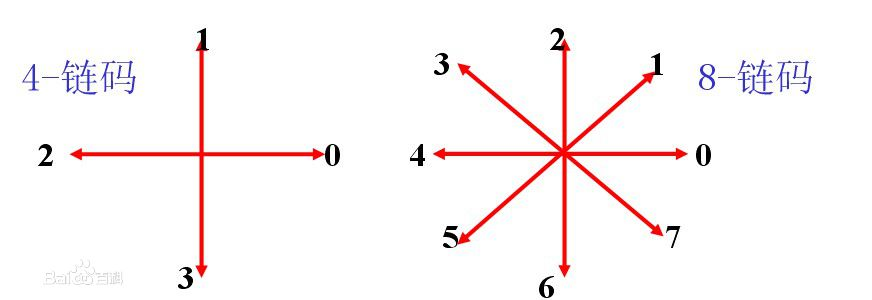用Freeman链码表示曲线时需要曲线的起点，对8链码而言，奇数码和偶数码的对应线段长度不等，规定偶数码单位长度为1，奇数码的单位长度为根号2.

1、原链码

2、归一化链码

3、归一化差分码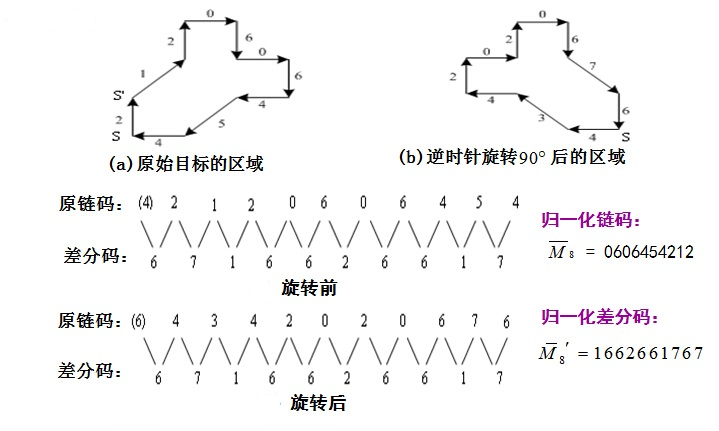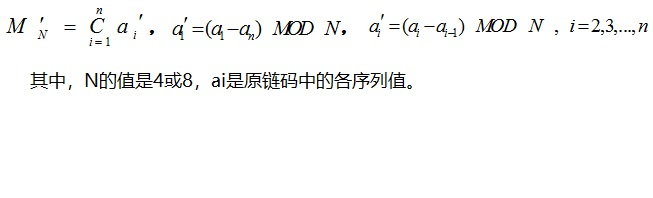：差分码中a(i)-a(i-1)是暗战顺时针的方向计算两个数之间的间隔。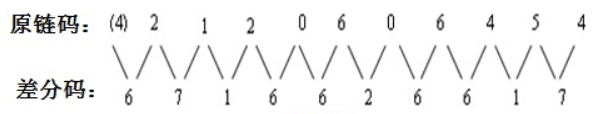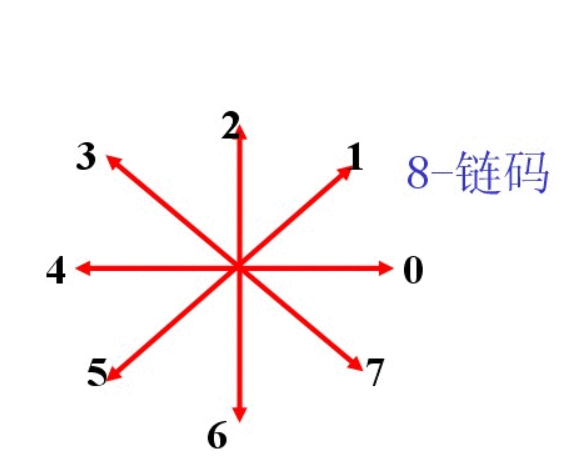6的得到是2->4按照8链码顺时针经过的间隔 2->1->0->7->6->5->4 共6个间隔。

2->1 经过 2->1 共1个间隔。

2018-08-17 09:15:30 zcg1942 阅读数 1189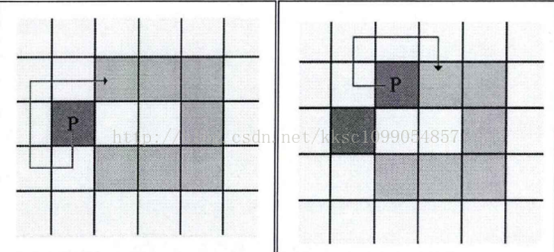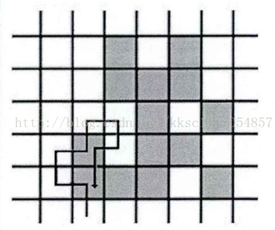3 2 1 4 0 5 6 7

Freeman链码显然与初始点的选取有关，我们还需要使编码与初始锚点无关，即无论选择哪一个点做起始点，最后的编码都相同。注意到如果依次使用不同的点做初始点，编码之间的关系是循环移位，于是我们可以对它们进行归一化，选择其中最小的最为最终的编码（把编码看作自然数进行大小的比较）。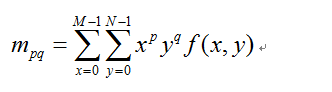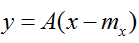A由x的协方差矩阵的特征向量构成。y的协方差矩阵是对角矩阵，主对角线的特征值就是x的协方差矩阵的特征值。可以通过y重构x，当不使用所有的特征值和其对应的特征向量重构时，误差就是剩余没有使用的特征值的和，所以我们霍特林变换是均方误差最小的，因为A的行向量对应的特征值是递减的。霍特林变换也叫主分量变换。

Reference：

1. Square：https://blog.csdn.net/kksc1099054857/article/details/74937731
2. Freeman：https://blog.csdn.net/yang6464158/article/details/39801381